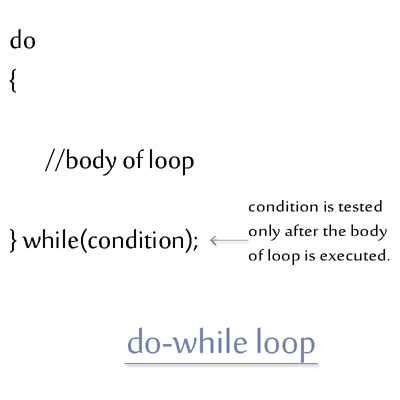< Prev
Next >

# C - Do While Loop

In do-while loop of C language, a block of statements or the body of loop always comes before the condition of do-while loop is tested. This is the only looping construct which allows us to execute its associated block of statements(atleast once), irresepective of the result of its boolean condition. Let's us see the working of do-while loop.

## Working of do-while loop

• During the first run of do-while loop, the body of do-while loop is always executed, even when the its underneath condition of do-while loop is false.

• Next, if this condition of do-while loop is true, only then its body of loop in next run of do-while loop is executed and the loop continues.

• But, if this condition of do-while loop is false, then its body of loop in the next run of do-while loop is not executed and the loop terminates.

## Note :

The body of do-while loop is always executed in its first run, even when the condition of do-while loop is false.## do while loop example

In this example, we are going to print numbers from 0 to 5 using a do-while loop.

``````/* do-while example */

#include<stdio.h>
int main()  /* main method starts */
{
int i=0;

do
{

printf("i = %d \n",i);
i++;

}while(i<5);

return 0;
}
``````

## Output

``````i = 0
i = 1
i = 2
i = 3
i = 4``````

## do while loop is executed at least once

The body of do while loop is always executed during its first execution, even when the condition of loop is evaluated to be false, take a look at our next example -

``````/* do-while second example */

#include<stdio.h>

int main()  /* main method starts */
{
int i=100;

do
{

printf("i = %d \n", i);
i++;

}while(i<10);

return 0;
} /* main method ends and so does the program */
``````

## Output

``i = 100``

< Prev
Next >
< For Loop
While Loop>